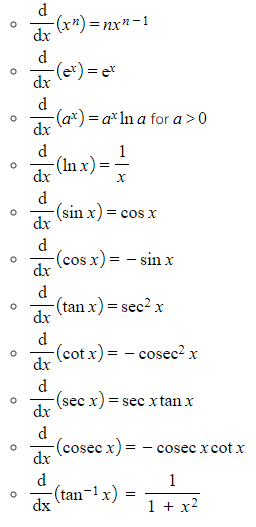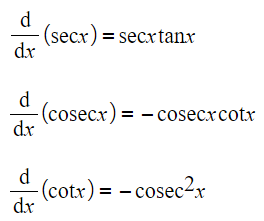# CIE A Level Maths: Pure 3复习笔记4.1.1 Differentiating Other Functions (Trig, ln & e etc)

### Differentiating Other Functions (Trig, ln & e etc)

#### How do I differentiate common functions?

• These are the common results

####How do I differentiate exponentials and logarithms?

• The two basic differentiation formulae are:• From those basic formulae are derived these two additional formulae:• And for exponentials more generally:• This last formula can be derived from Formula 3 by using the chain rule

#### How do I differentiate the reciprocal trigonometric functions?

• The formulae for the derivatives of the reciprocal trigonometric functions are:• You can derive the derivatives for seccosec, and cot using the chain rule and the derivatives of the basic trigonometric functions#### Exam Tip

• All of the above are in the formula book, make sure you know how to find them.
• They will be particularly useful when working with identities and when integrating trigonometric functions.
• Recognising the derivative of tan-1x will be particularly useful for integrating some functions.

#### Worked Example